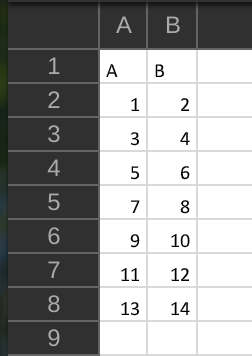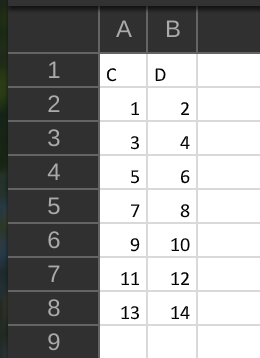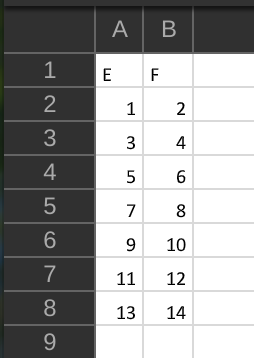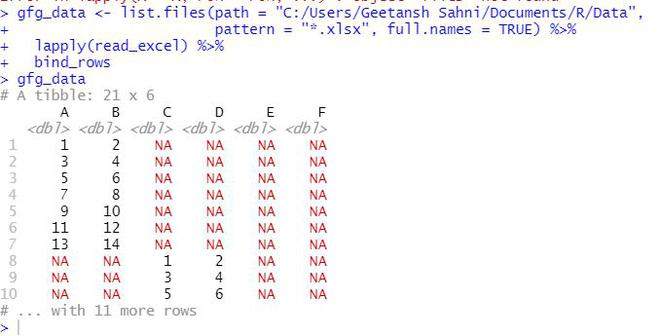Open In App

# How to read multiple Excel files in R

In this article, we will discuss how to merge multiple Excel files in the R programming language.

## Modules Used:

• dplyr: The dplyr package in R is a structure of data manipulation that provides a uniform set of verbs, helping to resolve the most frequent data manipulation hurdles.
• plyr: The “plyr” package in R is used to work with data, including its enhancements and manipulations.
• readxl: This package is used to work with excel files in R

## Functions Used:

• list.files() function produces a character vector of the names of files or directories in the named directory.

Syntax: list.files(path = “.”, pattern = NULL, all.files = FALSE,full.names = FALSE, recursive = FALSE, ignore.case = FALSE, include.dirs = FALSE, no.. = FALSE)

• lapply() function returns a list of the same length as X, each element of which is the result of applying FUN to the corresponding element of X.

Syntax: lapply(X, FUN, …)

• bind_rows() function is an efficient implementation of the common pattern of do.call(rbind, dfs) or do.call(cbind, dfs) for binding many data frames into one.

Syntax: bind_rows(…, .id = NULL)

Files in use:gfg_data1.xlsxgfg_data2.xlsxgfg_data3.xlsx

Example: Merging Excel using R

## R

 `library``(``"dplyr"``)                                                ``library``(``"plyr"``)                                                 ``library``(``"readr"``)  ``library``(``"readxl"``)`` ` `gfg_data <- ``list.files``(path = ``"Location/to/folder"``,    ``                       ``pattern = ``"*.xlsx"``,``                       ``full.names = ``TRUE``) %>% ``  ``lapply``(read_excel) %>%                                           ``  ``bind_rows                                                      `` ` `gfg_data                                                         `

Output: